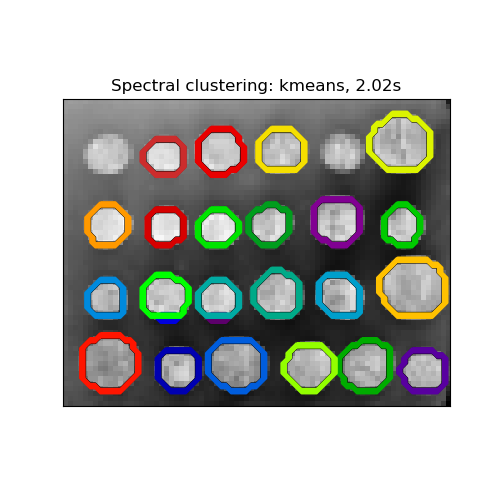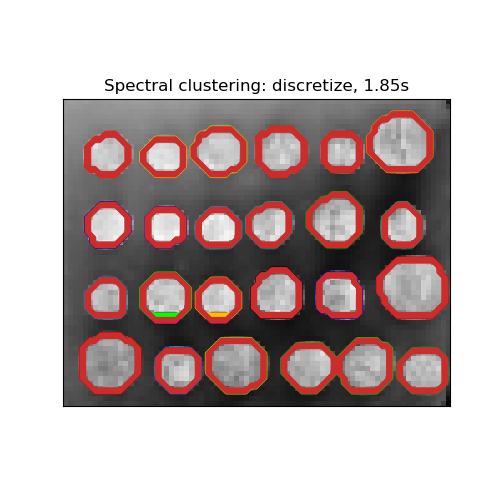# Segmenting the picture of greek coins in regions¶

This example uses Spectral clustering on a graph created from voxel-to-voxel difference on an image to break this image into multiple partly-homogeneous regions.

This procedure (spectral clustering on an image) is an efficient approximate solution for finding normalized graph cuts.

There are two options to assign labels:

• with ‘kmeans’ spectral clustering will cluster samples in the embedding space using a kmeans algorithm

• whereas ‘discrete’ will iteratively search for the closest partition space to the embedding space.

print(__doc__)

# Author: Gael Varoquaux <gael.varoquaux@normalesup.org>, Brian Cheung

import time

import numpy as np
from distutils.version import LooseVersion
from scipy.ndimage.filters import gaussian_filter
import matplotlib.pyplot as plt
import skimage
from skimage.data import coins
from skimage.transform import rescale

from sklearn.feature_extraction import image
from sklearn.cluster import spectral_clustering

# these were introduced in skimage-0.14
if LooseVersion(skimage.__version__) >= '0.14':
rescale_params = {'anti_aliasing': False, 'multichannel': False}
else:
rescale_params = {}

# load the coins as a numpy array
orig_coins = coins()

# Resize it to 20% of the original size to speed up the processing
# Applying a Gaussian filter for smoothing prior to down-scaling
# reduces aliasing artifacts.
smoothened_coins = gaussian_filter(orig_coins, sigma=2)
rescaled_coins = rescale(smoothened_coins, 0.2, mode="reflect",
**rescale_params)

# Convert the image into a graph with the value of the gradient on the
# edges.
graph = image.img_to_graph(rescaled_coins)

# Take a decreasing function of the gradient: an exponential
# The smaller beta is, the more independent the segmentation is of the
# actual image. For beta=1, the segmentation is close to a voronoi
beta = 10
eps = 1e-6
graph.data = np.exp(-beta * graph.data / graph.data.std()) + eps

# Apply spectral clustering (this step goes much faster if you have pyamg
# installed)
N_REGIONS = 25


Visualize the resulting regions

for assign_labels in ('kmeans', 'discretize'):
t0 = time.time()
labels = spectral_clustering(graph, n_clusters=N_REGIONS,
assign_labels=assign_labels, random_state=42)
t1 = time.time()
labels = labels.reshape(rescaled_coins.shape)

plt.figure(figsize=(5, 5))
plt.imshow(rescaled_coins, cmap=plt.cm.gray)
for l in range(N_REGIONS):
plt.contour(labels == l,
colors=[plt.cm.nipy_spectral(l / float(N_REGIONS))])
plt.xticks(())
plt.yticks(())
title = 'Spectral clustering: %s, %.2fs' % (assign_labels, (t1 - t0))
print(title)
plt.title(title)
plt.show()

••Out:

Spectral clustering: kmeans, 2.02s
Spectral clustering: discretize, 1.85s


Total running time of the script: ( 0 minutes 4.687 seconds)

Estimated memory usage: 9 MB

Gallery generated by Sphinx-Gallery